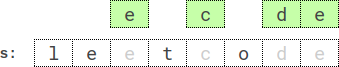##### Welcome to Subscribe On Youtube

Formatted question description: https://leetcode.ca/all/2030.html

# 2030. Smallest K-Length Subsequence With Occurrences of a Letter (Hard)

You are given a string s, an integer k, a letter letter, and an integer repetition.

Return the lexicographically smallest subsequence of s of length k that has the letter letter appear at least repetition times. The test cases are generated so that the letter appears in s at least repetition times.

A subsequence is a string that can be derived from another string by deleting some or no characters without changing the order of the remaining characters.

A string a is lexicographically smaller than a string b if in the first position where a and b differ, string a has a letter that appears earlier in the alphabet than the corresponding letter in b.

Example 1:

Input: s = "leet", k = 3, letter = "e", repetition = 1
Output: "eet"
Explanation: There are four subsequences of length 3 that have the letter 'e' appear at least 1 time:
- "lee" (from "leet")
- "let" (from "leet")
- "let" (from "leet")
- "eet" (from "leet")
The lexicographically smallest subsequence among them is "eet".


Example 2:Input: s = "leetcode", k = 4, letter = "e", repetition = 2
Output: "ecde"
Explanation: "ecde" is the lexicographically smallest subsequence of length 4 that has the letter "e" appear at least 2 times.


Example 3:

Input: s = "bb", k = 2, letter = "b", repetition = 2
Output: "bb"
Explanation: "bb" is the only subsequence of length 2 that has the letter "b" appear at least 2 times.


Constraints:

• 1 <= repetition <= k <= s.length <= 5 * 104
• s consists of lowercase English letters.
• letter is a lowercase English letter, and appears in s at least repetition times.

Similar Questions:

## Solution 1.

// OJ: https://leetcode.com/problems/smallest-k-length-subsequence-with-occurrences-of-a-letter/
// Time: O(N)
// Space: O(N)
class Solution {
public:
string smallestSubsequence(string s, int k, char ch, int rep) {
int N = s.size(), popped = 0, used = 0, cnt = 0;
for (char c : s) cnt += c == ch;
string ans;
for (int i = 0; i < N; ++i) {
// N - i -> remaining chars to be pushed
// k - (int)ans.size() + 1 -> the open space left in answer after popping
while (ans.size() && N - i >= k - (int)ans.size() + 1) {
bool pop;
if (s[i] == ch) {
if (ans.back() == ch) {
pop = false; // never pop
} else {
pop = ans.back() > s[i] // previous letter is greater
|| k - (int)ans.size() + used < rep; // if we don't pop, there is no space for ch
}
} else { // s[i] != ch
if (ans.back() == ch) {
pop = s[i] < ans.back() && cnt - popped - 1 >= rep;
} else {
pop = s[i] < ans.back();
}
}
if (!pop) break;
if (ans.back() == ch) ++popped, --used;
ans.pop_back();
}
used += s[i] == ch;
ans.push_back(s[i]);
}
while (ans.size() > k) ans.pop_back();
return ans;
}
};